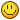## Recommended Posts

This function returns a random number with minimum min and maximum max with normal distribution. More info here

```public static int rand(int min, int max)
{
int n;
int mean = (min + max) / 2;
int std = (max - mean) / 3;
Random r = new Random();
do {
double val = r.nextGaussian() * std + mean;
n = (int) Math.round(val);
} while (n < min || n > max);
return n;
}
```
Edited by SESH
##### Share on other sites

I love this, I've been planning on writing this.

Glad I found your thread man, well done•1
##### Share on other sites

Not all that great, since that while loop can potentially block forever...

```    private final Random random = new Random();

public int exclusive(int min, int max) {
if (max <= min) {
max = min + 1;
}
return random.nextInt((max - min)) + min;
}

public int exclusive(int range) {
return exclusive(0, range);
}

public int inclusive(int min, int max) {
if (max < min) {
max = min + 1;
}
return exclusive((max - min) + 1) + min;
}

public int inclusive(int range) {
return inclusive(0, range);
} ```
##### Share on other sites

Not all that great, since that while loop can potentially block forever...

```    private final Random random = new Random();

public int exclusive(int min, int max) {
if (max <= min) {
max = min + 1;
}
return random.nextInt((max - min)) + min;
}

public int exclusive(int range) {
return exclusive(0, range);
}

public int inclusive(int min, int max) {
if (max < min) {
max = min + 1;
}
return exclusive((max - min) + 1) + min;
}

public int inclusive(int range) {
return inclusive(0, range);
} ```

Well what you did there is too complicated, what you can do is this:

```static Random r = new Random();
public static int rand (int mean, int stdDev){
return (int) (r.nextGaussian()*stdDev+mean);
}
```
##### Share on other sites

Well what you did there is too complicated, what you can do is this:

```static Random r = new Random();
public static int rand (int mean, int stdDev){
return (int) (r.nextGaussian()*stdDev+mean);
}
```

How is what I did complicated? I gave methods that support both inclusive and exclusive intervals.

##### Share on other sites

Not all that great, since that while loop can potentially block forever...

```    private final Random random = new Random();

public int exclusive(int min, int max) {
if (max <= min) {
max = min + 1;
}
return random.nextInt((max - min)) + min;
}

public int exclusive(int range) {
return exclusive(0, range);
}

public int inclusive(int min, int max) {
if (max < min) {
max = min + 1;
}
return exclusive((max - min) + 1) + min;
}

public int inclusive(int range) {
return inclusive(0, range);
} ```

It would be incredibly, incredibly unlikely for it to even run through the while loop twice.

Edited by SESH

## Join the conversation

You can post now and register later. If you have an account, sign in now to post with your account.×   Pasted as rich text.   Paste as plain text instead

Only 75 emoji are allowed.